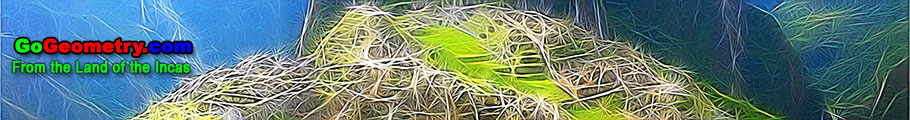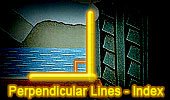Perpendicular Lines, Theorems and Problems - Table of Content  (20)Proposed Problem 62: Square Diagonal, Inscribed Circle, Perpendicular lines, Parallel lines. Sangaku Geometry Theorem: Cyclic Quadrilateral. Inradii, Rectangle, Perpendicular lines, Parallel lines. Interactive Hinged tessellation. Square tessellation - Dynamic Geometry. Please be patient while the applet loads on your computer. This Java applet requires Java 1.3 or higher. Perpendicular lines, Parallel lines. Triangle and Squares 22 Perpendicular lines, Parallel lines. Triangle and Squares 20 Perpendicular lines, Parallel lines. Proposed Problem 49. Angles and right triangle, Perpendicular lines. Triangle and Squares 17, Perpendicular lines. Problem 39. Triangle, Incircle, Bisector, Cyclic Quadrilateral and angles, Perpendicular lines. Proposed Problem 29. Right Triangle, altitude, incircle and inradius, Perpendicular lines. Ten conclusions. Proposed Problem 27 Right Triangle, incircles and inradius, Perpendicular lines. Eyeball Theorem: Animated Angle to Geometry Study. Perpendicular lines, Parallel lines. Proposed problem 20 Right Triangle, Perpendicular lines, Parallel lines, incircles and inradii. Parallelogram with Squares theorem Thébault's Theorem. Perpendicular lines Kurschak's Tile and Theorem. Jozsef Kurschak (Hungary, 1864-1933) An elegant and a purely geometric way of finding the area of a regular dodecagon. Euclid's Elements Book I, 23 Definitions. One-page visual illustration. Euclid's Elements Book. Index Go to Page: Previous| 1 | 2 | 3 | 4 | 5 | 6 | 7 | 8 | 9 | 10 | 11 | 12 | 13 | 14 | 15 | 16 | 17 | 18 | 19 | 20 | Next